# Complete system

(diff) ← Older revision | Latest revision (diff) | Newer revision → (diff)

closed system (of differential equations)

A system of first-order partial differential equations: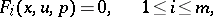(1)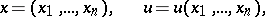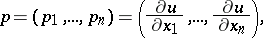with the following property: For any set of numberssatisfying (1), the equation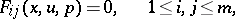is valid, whereare the Jacobi brackets.

The completeness condition may be formulated somewhat differently for a linear homogeneous system. The Jacobi brackets in that case are linear in the variables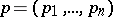; if the system is written in the form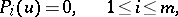where the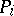are linear first-order differential operators, then these brackets correspond to the commutators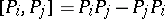. The system is complete if all commutators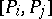can be expressed as linear combinations of the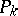's with coefficients depending only on.

If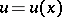is the joint solution of the two equationsthenis also a solution of the equation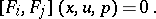(2)

An arbitrary system of the form (1) is usually extended to a complete one by adding new independent equations to it that have been obtained from the old ones by means of the formation of Jacobi brackets. In this extension, in accordance with (2), none of the solutions will be lost if the system is generally solvable.

A property of the system is that it is completely invariant under those non-singular transformations of the variablesfor which the meaning of the differential equations is retained. These transformations include, for example, diffeomorphisms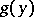,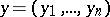, and also transformations of the following type. Let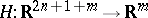be a smooth mapping such that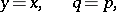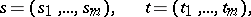is a diffeomorphism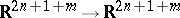. This transforms the system (1) into a system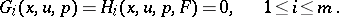How to Cite This Entry:
Complete system. Encyclopedia of Mathematics. URL: http://encyclopediaofmath.org/index.php?title=Complete_system&oldid=18756
This article was adapted from an original article by A.P. Soldatov (originator), which appeared in Encyclopedia of Mathematics - ISBN 1402006098. See original article